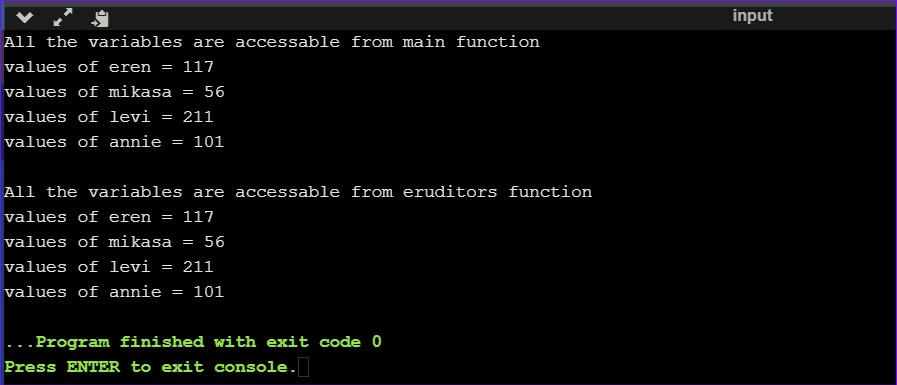What is Variable ?

Variable is a data name which is used to store some data value or symbolic names for storing program computations and results. Variable is a way to represent memory location to be easily identified.

Rules for naming Variables :

1. First character must be started with a letter or an underline not with a digit. The remaining are of an alphabet, any numeric digit or the underscore character.
2. Variable names must be unique.
3. Blank spaces and commas are not allowed within a Variable.
4. Keywords can't be used as Variable.
5. Variable type can be int, char, float, etc.
6. Variables are case sensitive. A variable name can consist of 52 alphabetic characters (both upper and lower case), the underscore character ( _ ) and 10 digits (i.e., 0-9).

Declaration of Variable :

Variables must be declared before they are used in the program. During the declaration, memory space is not allocated for the variable. It only happens during the variable definition(assigning variable in a memory location).

Reason behind variable declaration :
1. To inform the compiler about the variable's name.
2. To specify the datatype of the variable.

Syntax :
datatype variableName;

Example :
int anik,she;
char rias, mizuhara;

//Here, int and char are datatypes.
//Anik, she, rias, mizuhara are variable's name.

Variable Initialization :

Variable initialization is nothing but assigning a value to the variable.

Syntax :
datatype variableName = value;

Example :
int deb = 91;
float arijit = 211.169;
char sovan = 'K';

//Here, int, float and char are datatypes.
//91, 211.169, K are value assigned to the variables.

Types of Variables :

C programming language consists of 2 types of variables. They are :
1. Local Variable
2. Global Variable

1. Local Variable :

A variable which is declared inside the function is known as Local Variable. These variables are declared within the function and cannot be accessed outside the function. Local variables must have to be initialized before it's use.

Example :
#include<iostream>
using namespace std;
void eruditors();
int main()
{
int aqua = 56, kazuma = 117; // aqua and kazuma are local variables of main function. These variables are not visible to eruditors funtion.
// accessing lucy and natsu in this function will throw 'lucy' and 'natsu' are undeclared.

cout<<"values of aqua = "<<aqua <<endl <<"values of kazuma = " <<kazuma;
eruditors();
}

void eruditors()
{
int lucy = 47, natsu = 91; // lucy and natsu are local variables of test function.
// accessing aqua and kazuma in this function will throw 'aqua' and 'kazuma' are undeclared.

cout<<"\nvalues of lucy = "<<lucy <<endl <<"values of natsu = " <<natsu;
}

Output :Keypoints of Local Variable :

~ By default, local variables are uninitialized and it contains garbage value.
~  It is visible only inside their function, and only function's inside statements can access that local variable.
~ It is declared when control enters a function and gets destroyed, when control exits from function.

2. Global Variable :

A variable which is declared outside the function is known as Global Variable. These variables are defined outside the main function and so it can be accessed from anywhere in the function. Global variables must have to be declared before its' use.

Example :
#include<iostream>
using namespace std;
void eruditors();
int eren = 117, mikasa = 56;
int levi = 211, annie = 101;

//eren, mikasa, levi, annie all are global variables.

int main()
{
cout<<"All the variables are accessable from main function";
cout<<"\nvalues of eren = "<<eren <<endl <<"values of mikasa = "<<mikasa <<endl <<"values of levi = "<<levi <<endl <<"values of annie = "<<annie;
eruditors();
}

void eruditors()
{
cout<<"\n\nAll the variables are accessable from eruditors function";
cout<<"\nvalues of eren = "<<eren <<endl <<"values of mikasa = "<<mikasa <<endl <<"values of levi = "<<levi <<endl <<"values of annie = "<<annie;
}

Output :Keypoints of Global Variable :

~ Global variables are declared outside of any function.
~ Unlike local variable, global variables retain their values between function calls and throughout the program execution.
~ Global variables can be used by any piece of code as it is visible to every function.